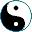Examples of Functors
<<
>>
 Identity functor - for a category X an identity X → X keeps objects snd arrows intact. Still it is a functor. Setf ↪ Set - a functor that includes Setf into Set, that is, maps each finite set to itself, and the same with functions. Note that this is not an identity functor. Set → Top - similar to the previous example, this functor makes Set a part of Top. Each set is mapped to a discrete topological space. For any set A we can define the following functor: (- xA): Set → Set - it will map any set X to a cartesian product, X × A. For any set A we can define a functor PA: Set → Set; it maps any set X to XA, a set of functions from A to X. Set ↪ Part embeds sets to sets with partial functions: it maps sets and functions to themselves. An opposite to 6. is a functor +Null: Part → Set -this functor adds an "extension" Null to each set: X ↦ (X+Null), so that a partial function X → Y maps to a function (X+Null) → (Y+Null). (Exercise. Define such an extension for partial functions.)